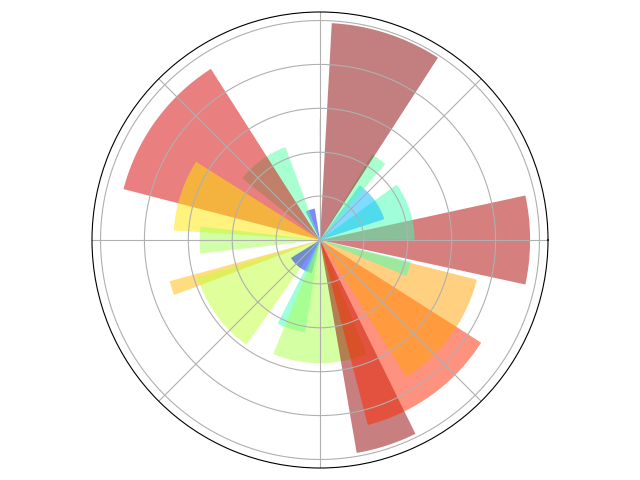# Plotting in polar coordinnates¶

A simple example showing how to plot in polar coordinnates with matplotlib.```import numpy as np
import matplotlib.pyplot as plt

ax = plt.axes([0.025, 0.025, 0.95, 0.95], polar=True)

N = 20
theta = np.arange(0.0, 2 * np.pi, 2 * np.pi / N)
width = np.pi / 4 * np.random.rand(N)
bars = plt.bar(theta, radii, width=width, bottom=0.0)